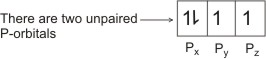## Electronic Configuration

Electronic configuration is the distribution of electrons in the shells and subshells of atoms of elements.

Each nth shell contains n subshells which consist of a total of n2 orbitals in which electrons are actually accommodated. For example, the first shell (K), n=1 contains one subshell which consists of one orbital. This orbital is the s orbital and is designated 1s (the 1 represents the value of n, which is the shell number or the principal quantum number).

Strictly speaking, the K shell is not divided into subshells. I.e., there is only one type of orbital, hence, the electrons in this shell are of the same energy. The 2nd shell (L), n=2 contains two subshells. One consists of one s orbital (designated as 2s), while the other consists of three p orbitals (each designated as 2p).

This brings the total number of orbitals in this shell to four. The 3rd shell (M), n=3 contains three subshells. The first consists of one s orbital (designated as 3s), the second consists of three p orbitals (each designated as 3p), and the third consists of five d orbitals (each designated as 3d). Thus , the total number of orbitals in this shell is nine. The 4th shell (N), n=4 contains for subshells.

The first consists of one s orbital (designated 4s), the second three p orbitals (each designated 4p), the third five d orbitals (each designated 4d), and the fourth seven f orbitals (each designated 4f). Thus the total number of orbitals in this shell is 16. Since each orbital can take a maximum of two electrons, and also for the fact that the number of orbitals in an nth shell is n2, the maximum number of electrons that can be accommodated in a shell is given as 2n2.

For example, the 1st shell (K), n= 1 can take a maximum of 2 electrons, the 2nd shell (L), n=2 can take 8, the 3rd shell (M), n=3 can take a maximum of 18 and the 4th shell (N) can take a maximum of 32 electrons.

Note: Within a given shell the electrons in the s orbital have the lowest energy, and the electrons in the f orbital have the highest energy, i.e., s< p< d < f.

In filling a set of energy levels (main energy or the orbitals), the lowest in energy is always filled first before the next – this is the Aufbau principle. I.e., K is filled before L, which is filled before M.

In the same way, s is filled first before p, which is filled before d.

All shells have an s orbital.

The energy of an electron in a given orbital in a particular shell is lower (i.e., the electrons are held more tightly) than that of an electron in an orbital of the same type in a shell with a higher number, i.e., 1s < 2s < 3s < 4s < 5s < 6s < 7s.

When filling a subshell, each orbital is first filled singly before pairing - this is the principle of maximum multiplicity, sometimes called Hund’s Rule.

For example: to fill the p subshell with 4 electrons:See examples of electronic configuration of atoms of different elements

 Like This Post? Please Share!!

Copyright , All Rights Reserved Free Chemistry Online | About Us | Usage of Content | Total Disclosures | Privacy Policy